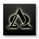Dilihat 3319
Instead of calculating Relative Strength ( RS ) of Price( RSI ), this calculates RS of Moving Average(RSIOMA).

Credit goes to Kalenzo from the Forex TSD forum http://www.forex-tsd.com/metatrader-4/82...

I easily modified the default RSI in trading view to make this indicator.
```//By Darkyalt
study(title="Relative Strength Index of MA", shorttitle="RSIOMA")
src = close, len = input(14, minval=1, title="Length")
up = rma(max(change(ema(src, len)), 0), len)
down = rma(-min(change(ema(src, len)), 0), len)
rsi = down == 0 ? 100 : up == 0 ? 0 : 100 - (100 / (1 + up / down))
plot(rsi, color=blue)
band1 = hline(80)
band0 = hline(20)
fill(band1, band0, color=purple, transp=90)
hline(50, color=gray, linestyle=line)
sig = ema(rsi, 21)
plot(sig, color=purple)```

## KomentarNice job. Thanks for sharing it with us. I presume we can try different MAs by substituting for ema. Have you tried any other variations?
JawabAlphaoptions
Thanks. I haven't tried any other type of moving averages because I like to keep things simple(K.I.S.S.) and I think the differences is negligible.

Feel free to try other MAs by substituting the ema in here to the MA you want.
#4 up = rma(max(change(ema(src, len)), 0), len)
#5 down = rma(-min(change(ema(src, len)), 0), len)

You can also change the signal(trigger) line and length here
#12 sig = ema(rsi, 21)
Jawab